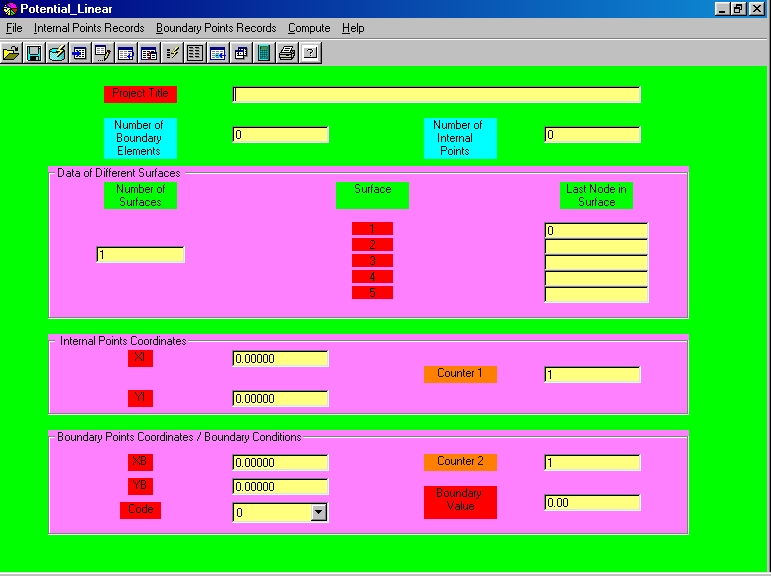Program 4: Potential Problems with Linear Elements (Potential_Linear)A. GENERAL INFORMATION

1. The current program is a result of the S.I.O.M. ( Singular Integral Operators Method ) for the solution of isotropic potential problems with linear elements.

2. The capabilities of this potential computer program is the computation of the potential function and potential derivative on the boundary and at any internal points.

3. The current program can be used for the solution of potential problems with up to five surfaces, like bodies with holes, etc.

B. SPECIAL INFORMATION

1. For external surfaces the numbering scheme is defined in the counterclockwise direction.

2. For internal surfaces the numbering scheme is defined in the clockwise direction.

3. Boundary Conditions. If code = 0 then the value of the potential at the node should be given.

4. Boundary Conditions. If code = 1 then the value of the potential derivative at the node should be given.

C. DATA ENTRY - OUTPUT RESULTS

1. The program opens a file *.dat (data entry). This file includes all the requested data.

2. The results of the program are given by a file *.res (output results).

3. Both data entry (file *.dat) and output results (file *.res) are printed by the program.

D. KEYS OF THE PROGRAM

The Records are referred to:

a. Internal Points (Coordinates).

b. Boundary Points (Coordinates and Boundary Conditions).

The keys are:

1. Add Records: Records are added by placing in Counter a number 1,2,3, ...etc.and then by pressing key "Add".

2. Edit Records: Records are edited by placing in Counter a number 1,2,3, ...etc and then by pressing key "Edit".

3. Erase Records: In order to erase a specific Coordinate, write in Counter its corresponding number and then press "Erase".

4. Insert Records: In order to insert a specific Coordinate between two other existing coordinates, write in Counter its corresponding number and then press "Insert".